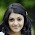# Computer System Architecture Set 12

### Questions 111 to 120

111.
What is represented by D7I'T in the instruction cycle?
 (a) Register – Reference Instructions (b) Program Interrupt (c) Input-Output Instructions (d) Branch Instruction (e) Memory Reference Instructions.
112.
What is the (r-1)’s complement of 345 in octal number system?
 (a) 342 (b) 234 (c) 243 (d) 432 (e) 423
113.
What is described by the Mnemonic SHRA?
 (a) Arithmetic shift left (b) Logical shift right (c) Logical shift left (d) Circular shift right (e) Arithmetic shift right.
114.
Which of the following terms describe that the information which will be used in near future is likely to be in use already?
 (a) Spatial Locality (b) Locality (c) Hit ratio (d) Temporal Locality (e) Effective access time.
115.
CISC stands for
 (a) Control Instruction Set Completeness (b) Complex Instruction Set Conversion

 (c) Complex Instruction Set Computer (d) Control Instruction Set Computer (e) Complex Instruction Set Control.
116.
What are the three state gates in a digital circuit?
 (a) Logic 0, Logic 1 Complement, High Impedance (b) Logic 0 Complement,  Logic 1, High Impedance (c) Logic 0 Complement, Logic 1 Complement, High Impedance (d) Logic 0, Logic 1, Low Impedance (e) Logic 0, Logic 1, High Impedance.
117.
What is the table that lists the required inputs for a given change of state?
 (a) Characteristic table (b) Truth table (c) Null table (d) Excitation table (e) Binary table.
118.
Microphone is a/an
 (a) Output device (b) Storage device (c) Input device (d) Processing device (e) Printing device.
119.
Of the following phases, identify the phase in which the programs must reside in the main memory.
 (a) Deletion (b) Execution (c) Printing (d) Reading (e) Insertion.
120.
(x')'=_____
 (a) x2 (b) 0 (c) 1 (d) x (e) x'.

#### Answers

 111 Answer :       (a) Reason:  D7I'T  in the instruction cycle represents Register –reference instructions. 112 Answer :       (d) Reason:  (r-1)’s complement of 345 in octal number system is 432. 113 Answer :       (e) Reason:  SHRA  stands for Arithmetic shift right. 114 Answer :       (d) Reason:  Temporal Locality describe that the information which will be used in near future is likely to be in use already. 115 Answer :       (c) Reason:  CISC stands for Complex Instruction Set Computer. 116 Answer :       (e) Reason:  The three state gates in a digital circuit Logic 0, logic 1, high impedance 117 Answer :       (d) Reason:  The table that lists the required inputs for a given change of state is Excitation Table. 118 Answer :       (c) Reason:  Microphone is a Input device. 119 Answer :       (b) Reason:  Execution is the phase in which the programs must reside in the main memory. 120 Answer :       (d) Reason:  (x')' = x.

29  30  31  32  33  34  35  36  37  38  39  Next >>

#### 1 comment :

1.Hi, Nice set of questions about Computer System Architecture.Thanks for support in helping me..

-Aparna
Theosoft